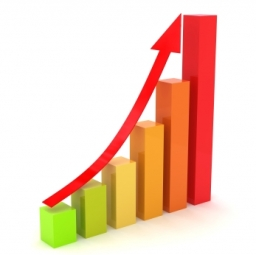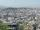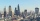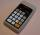# Percentage increase

What is the annual percentage increase in the city when the population has tripled in 20 years?

p =  5.6467 %

### Step-by-step explanation:Did you find an error or inaccuracy? Feel free to write us. Thank you!Tips to related online calculators
Do you want to convert time units like minutes to seconds?

## Related math problems and questions:

• DemographicsThe population grew in the city in 10 years from 30000 to 34000. What is the average annual percentage increase of population?
• Annual growthThe population has grown from 25,000 to 33,600 in 10 years. Calculate what was the average annual population growth in%?
• Exponential equationFind x, if 625 ^ x = 5 The equation is exponential because the unknown is in the exponential power of 625
• Annual increaseThe number of cars produced increased from 45,000 to 47,000 in 3 years. Calculate the average annual increase in cars in%.
• PopulationThe town has 65,000 inhabitants. 40 years ago, there were 157,000. How many people will live in a city in 10 years if the population's average rate is as in previous years?
• PopulationWhat is the population of the city with 3% annual growth, if in 10 years the city will have 60,000 residents?
• DecibelBy what percentage does the sound intensity increase if the sound intensity level increases by 1 dB?
• Volume of woodEvery year, at the same time, an increase in the volume of wood in the forest is measured. The increase is regularly p% compared to the previous year. If in 10 years the volume of wood has increased by 10%, what is the number p?
• Growth of woodThe annual growth of wood in the forest is estimated at 2%. In how many years will make the forest volume double?
• The city 3The city has 22,000 residents. How long it is expected to have 25,000 residents if the average annual population growth is 1.4%?
• Geometric sequenceIn the geometric sequence is a4 = 20 a9 = -160. Calculate the first member a1 and quotient q.
• The cityAt the end of 2010 the city had 248000 residents. The population increased by 2.5% each year. What is the population at the end of 2013?
• The townThe town population is 56000. It is decreasing by 2% every year. What will be the population of the town after 13 years?
• The city 2Today lives 298000 citizens in the city. How many citizens can we expect in 8 years if their annual increase is 2.4%?
• ExpIf sqrt(202-122) = sqrt[n](256), then n is:
• Car valueThe car loses value 15% every year. Determine a time (in years) when its price will halve.
• Profit growthThe profit of a company increased by 25% during the year 1992, increased by 40% during the year 1993, decreased by 20% in 1994 and increased by 10% during the year 1995. Find the average growth in the profit level over the four years periods?